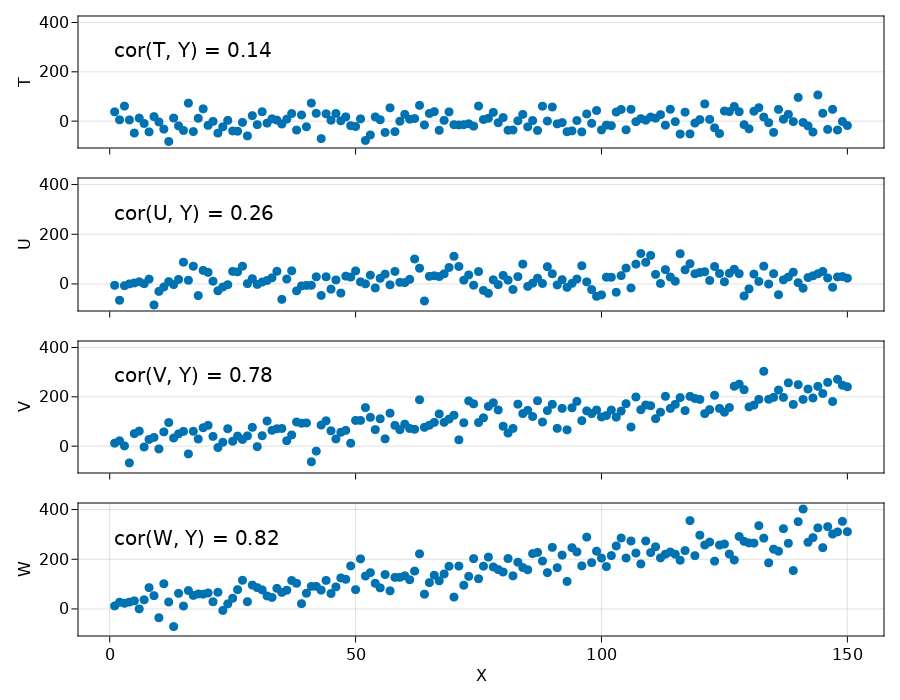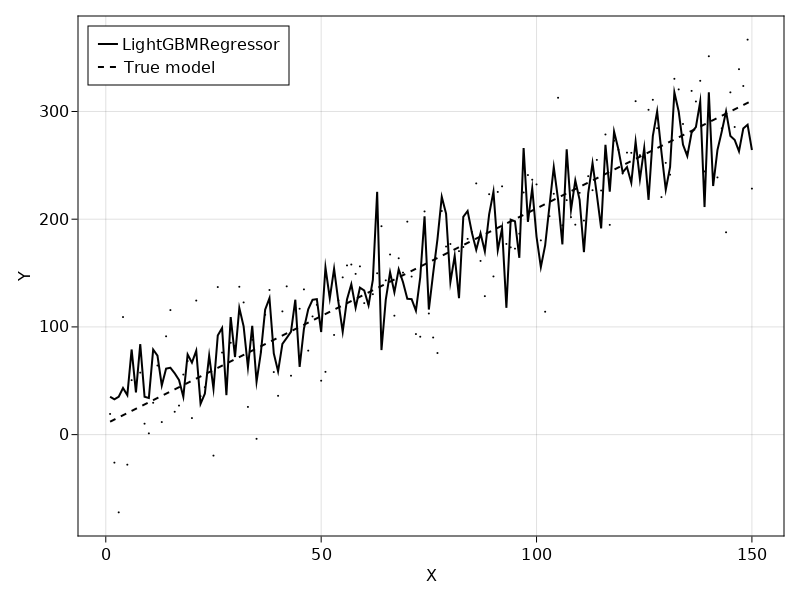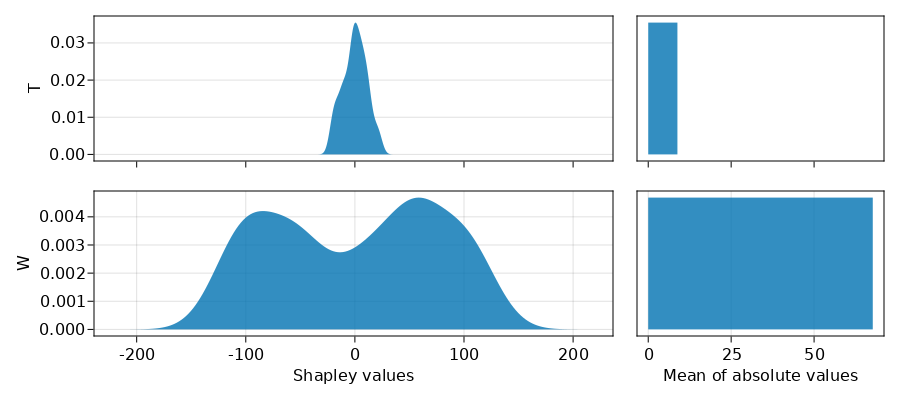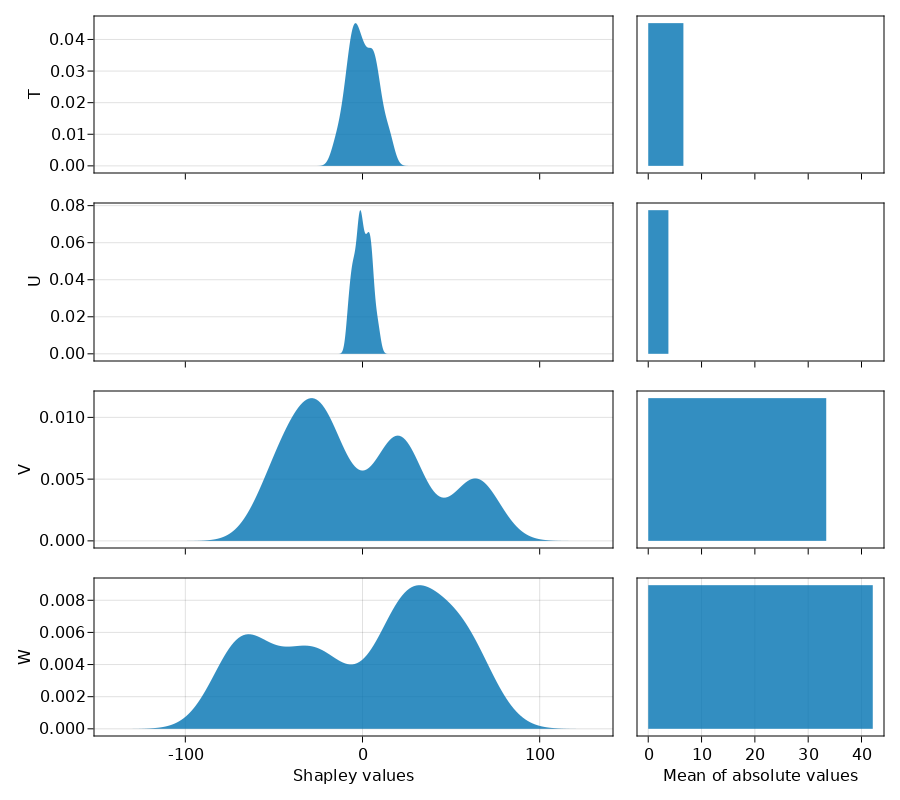Linear statistical models are great for many use-cases since they are easy to use and easy to interpret. Specifically, linear models can use features (also known as independent variables, predictors or covariates) to predict an outcome (also known as dependent variables).

In a linear model, a higher coefficient for a feature, the more a feature played a role in making a prediction. However, when variables in a regression model are correlated, these conclusions don't hold anymore.

One way to solve this is to use clustering techniques such as principal component analysis (PCA) (Dormann et al., 2012). With PCA, latent clusters are automatically determined. Unfortunately, these latent clusters now became, what I would like to call, magic blobs. Proponents of these techniques could say: "But we know that there is an underlying variable which causes our effect, why can't this variable be the same as the cluster that we found?" Well, because these blobs are found in the data and not in the real-world.

To link these blobs (officially, clusters) back to the real-world, one can try to find the features closes to the blobs in one way or another, but this will always introduce bias. Another approach is to drop features which are highly correlated and expected to be less important.

Some say that random forests combined with Shapley values can deal with collinearity reasonably well. This is because the random forest can find complex relations in the data and because Shapley values are based on mathematically proven ideas. Others say that the Shapley values will pick one of the correlated features and ignore the others. In this post, I aim to simulating collinear data and see how good the conclusions of the model are.

## Simulating data

``````begin
using CairoMakie
using DataFrames: Not, DataFrame, select
using Distributions: Normal
using LightGBM.MLJInterface: LGBMRegressor
using MLJ: fit!, machine, predict
using Random: seed!
using Shapley: MonteCarlo, shapley
using StableRNGs: StableRNG
using Statistics: cor, mean
end``````
``y_true(x) = 2x + 10;``
``y_noise(x, coefficient) = coefficient * y_true(x) + rand(Normal(0, 40));``
``indexes = 1.0:150.0;``
``r2(x) = round(x; digits=2);``
``````df = let
seed!(0)
X = indexes
T = y_noise.(indexes, 0)
U = y_noise.(indexes, 0.05)
V = y_noise.(indexes, 0.7)
W = y_noise.(indexes, 1)
Y = y_noise.(indexes, 1)

DataFrame(; X, T, U, V, W, Y)
end``````
X T U V W Y
1.0 37.7188 -5.61088 11.9561 12.1441 19.1759
2.0 5.35691 -65.7259 21.7467 27.0804 -26.0083
3.0 61.0028 -7.06661 1.05973 22.6588 -72.1792
4.0 4.95605 0.0830817 -68.0209 27.5124 109.146
5.0 -48.2309 3.72674 50.7662 32.4407 -27.9034
6.0 12.4727 8.59667 61.0154 0.215236 50.5563
7.0 -9.38565 0.888579 -3.47392 36.6952 42.0977
8.0 -43.4941 19.4709 27.1996 85.7672 57.5553
9.0 18.4924 -84.6015 35.4103 53.3868 10.1422
10.0 -3.22372 -30.0084 -11.2833 -35.4048 1.15114
...
150.0 -17.879 23.2258 240.651 310.904 228.384## Fitting a model

For the LGBM regressor, I've set some hyperparameters to lower values because the default parameters are optimized for large datasets.

``````function regressor()
kwargs = [
:num_leaves => 9,
:max_depth => 3,
:min_data_per_group => 2,
:learning_rate => 0.5
]
return LGBMRegressor( ; kwargs...)
end;``````
``````function fit_model(df::DataFrame)
X = select(df, Not([:X, :Y]))
y = df.Y
m = fit!(machine(regressor(), X, y))
return X, y, m
end;``````

To get an idea of what the model is doing, we can plot the predictions on top of the data.My main worry after seeing this is how we can avoid overfitting random forests on our data. Intuitively, it makes sense that the model overfits due to it's high flexibility combined with few samples. Of course, not all real-world phenomenons are linear, but it seems that the model is spending too much effort on fitting noise. Luckily, approaches such as (nested) cross-validation can estimate how well a model will generalize.

Anyway, I'm digressing. Back to the original problem of whether we can infer anything from the non-linear model about feature importance.

## Shapley values

Shapley values are based on a theory by Shapley (1953). The goal of these values is to estimate how much each feature has contributed to the prediction. One way to do this is by changing the input for a feature while keeping all other feature inputs constant and seeing how much the output changes. This repeated sampling is called the Monte Carlo method. We can aggregate the Monte Carlo results to estimate how much a feature contributes to the outcome.

``````function shapley_values(df::DataFrame)
X, y, m = fit_model(df)
mc = MonteCarlo(1024)
return shapley(x -> predict(m, x), mc, X)
end;``````

As a simple check, let's first see what happens if we only pass the dataset with X, T, W and Y. Because T has no relation to the outcome Y and W has a strong relation to the outcome Y, we expect that T gets a low score and W gets a high score.

In the plot below, I've shown all the Shapley values from the Monte Carlo simulation on the left and aggregated them on the right. As expected, the plots on the right clearly show that W has a greater contribution.

As a sidenote, usually people only show the plot on the right (mean of absolute values) when talking about Shapley values, but I think it is good to have the one on the left (Shapley values) too to give the full picture.Now, it's time for the final test: do Shapley values give appropriate credit to correlated features? We check this by passing all the features instead of only X, T, W and Y.Based on this outcome, I would say that Shapley values do a pretty good job in combination with the LightGBM model. The values T, U, V, W are ordered by their correlation with the outcome (and each other). As expected, features lower in the plot get a higher mean of absolute values, that is, feature importance. However, basing scientific conclusions on these outcomes may be deceptive because it's likely that there is a high variance on the reported feature importances. The main risk is here is that the model didn't properly fit resulting in incorrect outcomes. Another risk is that the overfitted model generalizes poorly. Both risks also hold for linear models, so I guess it's just the best we have.

# Conclusion

Based on this simple example, I would say that LightGBM combined with Shapley values can handle multicollinearity to some extend. Specifically, the outcomes mainly depend on whether the fitted model has used the less correlated features or decided to only use the highly correlated features. In this case, LightGBM has overfitted the data, which is probably why semi correlated features still receive some recognition even though other features are more useful. So, in this multicollinear case, an overfitted model gives better feature importance estimates. Doubts remain about generalizability.

Built with Julia 1.7.2 and

CairoMakie 0.7.2
DataFrames 1.3.2
Distributions 0.25.46
LightGBM 0.5.2
MLJ 0.17.1
Shapley 0.1.1
StableRNGs 1.0.0